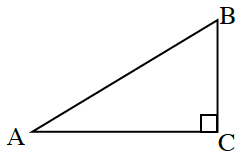### Home > INT3 > Chapter 2 > Lesson 2.2.1 > Problem2-47

2-47.

The right triangle shown at right has a height ($\overline { BC }$) of $12.0$ cm, and its area is $60.0$ square cm. What are $m∠B$ and the length of the hypotenuse?

Use $A=\frac{1}{2}bh$ and solve for $b$.

Use the answer from the previous hint to find the length of $\overline{AB}$.

Try using the Pythagorean Theorem.

Remember that  $\tan B=\frac{\text{opp}}{\text{adj}}$.

$m\measuredangle B=\tan^{-1}\frac{10}{12}\approx40^\circ$

$\sqrt{244}\approx15.6$ cm